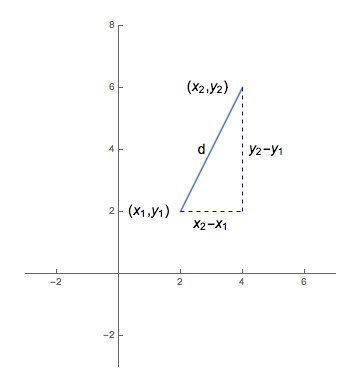## Distance, Circles Semi-Circles

### The Distance Formula

Suppose we are interested in finding the distance $d$ between two points $(x_1,y_1)$ and $(x_2,y_2)$.

Notice we can construct a right triangle by connecting the two points and drawing segments parallel to the two axes, as shown in the image below.Given that this is a right triangle, we can appeal to the Pythagorean Theorem to discover

$$(x_2-x_1)^2 + (y_2 - y_1)^2 = d^2$$

Taking the square root of both sides, without worrying about possible negative values as we are assured every such distance $d$ must be positive, we arrive at the distance formula:

$$d = \sqrt{(x_2-x_1)^2 + (y_2 - y_1)^2}$$

Note that in the expression on the right above, reversing the order of subtraction (i.e., replacing $x_2-x_1$ with $x_1-x_2$ and $y_2-y_1$ with $y_1-y_2$) does not alter the value of the expression. Doing so merely adds a couple of negative factors that get eliminated when the squaring happens. What is important is how far apart $x_1$ is from $x_2$ and how far apart $y_1$ is from $y_2$. That is to say, how much do the $x$ and $y$ coordinates change as we move from one point to the other?

With this in mind, recall that in mathematics and the sciences, one often uses a capital delta ($\Delta$) to represent some change in some quantity. We can do the same here, to rewrite the distance formula given above in a more easily remembered way: $$d = \sqrt{\Delta x^2 + \Delta y^2}$$

### Circles

Once we have access to the distance formula, finding the equation of a circle is almost trivial.

Think about the definition of a circle: "the set of all points $(x,y)$ at some fixed distance $r$ (called the radius) from some point $(h,k)$ (called its center)"

So the points of the circle are precisely those where the distance between $(x,y)$ and $(h,k)$ is $r$.

Written algebraically, we then have:

$$r = \sqrt{(x-h)^2 + (y-k)^2}$$

Simplifying things a bit by squaring both sides, we arrive at one of the more commonly seen forms for the equation of a circle:

$$(x-h)^2 + (y-k)^2 = r^2$$

We could, of course, expand the left side to get a polynomial that is quadratic in terms of both $x$ and $y$ (and where the coefficients on the $x^2$ and $y^2$ terms are both $1$. For example:

$$\begin{array}{rcl} (x-2)^2 + (y-3)^2 &=& 5 \quad \textrm{ is equivalent to...}\\\\ x^2 -4x + 4 + y^2 - 6y + 9 &=& 5 \quad \textrm{ and }\\\\ x^2 - 4x + y^2 - 6y + 8 &=& 0 \end{array}$$

This suggests another common form for the equation of a circle:

$$x^2 + ax + y^2 + by + c = 0$$

Of course to get from this form back to the first form, we just need to complete the square twice.

### Semi-circles

There can be no doubt that a circle is not a function -- it doesn't pass the vertical line test.

That said, what happens if we try to solve for $y$ as a function of $x$ in the equation for a circle? Consider the calculations below.

$$\begin{array}{rcl} (x-h)^2 + (y-k)^2 &=& r^2\\\\ (y-k)^2 &=& r^2 - (x-h)^2\\\\ y-k &=& \pm \sqrt{r^2 - (x-h)^2}\\\\ y &=& k \pm \sqrt{r^2 - (x-h)^2} \end{array}$$

Given the "$\pm$" above, we can see that a circle can be algebraically split into two functions:

$$y = k + \sqrt{r^2 - (x-h)^2} \quad \textrm{and} \quad y = k - \sqrt{r^2 - (x-h)^2}$$

Recalling that the center of the circle occurs at $(h,k)$, and thus half the circle lies above $y=k$ and half the circle lies below $y=k$, along with the fact that every $y \ge k$ for every $y$ value in the first equation and $y \le k$ for every $y$ value in the second -- we see that the two equations above give the equations of semi-circles. In particular, these are the equations of the top and bottom halves of the related circle, respectively.

Had we solved for $x$ as a function of $y$ instead, we would have similarly arrived at the equations for the left and right halves of the related circle:

$$x = h - \sqrt{r^2 - (y-k)^2} \quad \textrm{and} \quad x = h + \sqrt{r^2 - (y-k)^2}$$